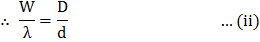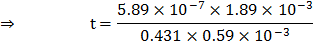# If fringes widthcm is 0.431 mm and shift of white central fringe on introducing a mica sheet in one path is 1.89 mm.  Thickness of the mica sheet will bea)b)c)d) None of these

## Question ID - 150180 :- If fringes widthcm is 0.431 mm and shift of white central fringe on introducing a mica sheet in one path is 1.89 mm.  Thickness of the mica sheet will bea)b)c)d) None of these

3537

(a)

When a mica sheet is introduced in the path of one of the two interfering beams, then entire fringe pattern is displaced towards the beam is the path of which plate is introduce, but fringe width is not changed.Also fringe width isAlso fringe width isUsing Eq. (ii) we get Eq. (i) asGiven,Next Question :
 A plane electromagnetic wave travelling along the-direction has a wavelength of. The variation in the electric field occurs in the-direction with an amplitude. The equations for the electric and magnetic fields as a function ofandare respectively a)b)c)d)